# cwtfilterbank

Continuous wavelet transform filter bank

## Description

Use `cwtfilterbank` to create a continuous wavelet transform (CWT) filter bank. The default wavelet used in the filter bank is the analytic Morse (3,60) wavelet. You can vary the time-bandwidth and symmetry parameters for the Morse wavelets, to tune the Morse wavelet for your needs. You can also use the analytic Morlet (Gabor) wavelet or bump wavelet. When analyzing multiple signals in time-frequency, for improved computational efficiency, you can precompute the filters once and then pass the filter bank as input to `cwt`. With the filter bank, you can visualize wavelets in time and frequency. You can also create filter banks with specific frequency or period ranges, and measure 3-dB bandwidths. You can determine the quality factor for the wavelets in the filter bank.

## Creation

### Syntax

``fb = cwtfilterbank``
``fb = cwtfilterbank(Name=Value)``

### Description

example

````fb = cwtfilterbank` creates a continuous wavelet transform (CWT) filter bank `fb`. The filters are normalized so that the peak magnitudes for all passbands are approximately equal to 2. The default filter bank is designed for a signal with 1024 samples. The default filter bank uses the analytic Morse (3,60) wavelet. The filter bank uses the default scales: approximately 10 wavelet bandpass filters per octave (10 voices per octave). The highest-frequency passband is designed so that the magnitude falls to half the peak value at the Nyquist frequency.As implemented, the CWT uses L1 normalization. With L1 normalization, equal amplitude oscillatory components at different scales have equal magnitude in the CWT. L1 normalization provides a more accurate representation of the signal. The amplitudes of the oscillatory components agree with the amplitudes of the corresponding wavelet coefficients. See Sinusoid and Wavelet Coefficient Amplitudes.`fb` can be used as input for `cwt`.```

example

````fb = cwtfilterbank(Name=Value)` creates a CWT filter bank `fb` with Properties using one or more name-value arguments. Properties can be specified in any order as `Name1=Value1,...,NameN=ValueN`. NoteYou cannot change a property value of an existing filter bank. For example, if you have a filter bank `fb` with a `SignalLength` of 2000, you must create a second filter bank `fb2` to process a signal with 2001 samples. You cannot assign a different `SignalLength` to `fb`. ```

## Properties

expand all

Length of the signal, specified as a positive integer. The signal must have at least four samples.

Example: `fb = cwtfilterbank(SignalLength=1700)`

Data Types: `double`

Analysis wavelet used in the filter bank, specified as `"Morse"`, `"amor"`, or `"bump"`. These strings specify the analytic Morse, Morlet (Gabor), and bump wavelet, respectively. The default wavelet is the analytic Morse (3,60) wavelet.

By default, for Morse wavelets, the frequency response decays to 50% of the peak magnitude at the Nyquist. For the Morlet and bump wavelets, the frequency response decays to 10% of the peak magnitude. You can change the decay percentage by setting the filter bank `FrequencyLimits` property. See `cwtfreqbounds`.

For Morse wavelets, you can also parameterize the wavelet using the `TimeBandwidth` or `WaveletParameters` properties.

Example: ```fb = cwtfilterbank(SignalLength=1700,wavelet="bump")```

Number of voices per octave to use for the CWT, specified as an integer between 1 and 48. The CWT scales are discretized using the specified number of voices per octave. The energy spread of the wavelet in frequency and time automatically determines the minimum and maximum scales.

You can use `cwtfreqbounds` to determine the frequency limits of the wavelet filter bank. The frequency limits depend on parameters such as the energy spread of the wavelet, number of voices per octave, signal length, and sampling frequency.

Data Types: `single` | `double`

Sampling frequency in hertz, specified as a positive scalar. If unspecified, frequencies are in cycles/sample and the Nyquist frequency is ½. To specify scales in periods, use the `SamplingPeriod` and `PeriodLimits` name-value arguments.

You cannot specify both the `SamplingFrequency` and `SamplingPeriod` properties.

Example: ```fb = cwtfilterbank(SamplingFrequency=5,wavelet="amor")```

Data Types: `single` | `double`

Frequency limits of the wavelet filter bank, specified as a two-element vector with positive strictly increasing entries.

• The first element specifies the lowest peak passband frequency. The frequency must be greater than or equal to the product of the wavelet peak frequency in hertz and two time standard deviations divided by the signal length.

• The second element specifies the highest peak passband frequency. The high frequency limit must be less than or equal to the Nyquist.

• The base-2 logarithm of the ratio of the high frequency limit, `fMax`, to the low frequency limit, `fMin`, must be greater than or equal to `1/NV`, where `NV` is the number of voices per octave:

```log2(fMax/fMin) ≥ 1/NV```.

If you specify frequency limits outside the permissible range, `cwtfilterbank` truncates the limits to the minimum and maximum values. Use `cwtfreqbounds` to determine frequency limits for different parametrizations of the wavelet transform.

If using a sampling period in the filter bank, you cannot specify the `FrequencyLimits` property.

Example: If ```fb = cwtfilterbank(SignalLength=1000,SamplingFrequency=1000,FrequencyLimits=[90 100])```, then ```log2(100/90) ≥ 1/fb.VoicesPerOctave```.

Data Types: `double`

Sampling period, specified as a duration scalar. You cannot specify both the `SamplingFrequency` and `SamplingPeriod` properties.

Example: ```fb = cwtfilterbank(SamplingPeriod=seconds(0.5))```

Data Types: `duration`

Period limits of the wavelet filter bank, specified as a two-element `duration` array with positive strictly increasing entries.

• The first element of `PeriodLimits` specifies the largest peak passband frequency and must be greater than or equal to twice the `SamplingPeriod`.

• The maximum period cannot exceed the signal length divided by the product of two time standard deviations of the wavelet and the wavelet peak frequency.

• The base-2 logarithm of the ratio of the minimum period, `minP`, to the maximum period, `maxP`, must be less than or equal to `-1/NV`, where `NV` is the number of voices per octave:

```log2(minP/maxP) ≤ -1/NV```.

If you specify period limits outside the permissible range, `cwtfilterbank` truncates the limits to the minimum and maximum values. Use `cwtfreqbounds` to determine period limits for different parametrizations of the wavelet transform.

If using a sampling frequency in the filter bank, you cannot specify the `PeriodLimits` property.

Example: If ```fb = cwtfilterbank(SignalLength=1000,SamplingPeriod=seconds(0.1),PeriodLimits=[seconds(0.2) seconds(3)])```, then ```log2(0.2/3) ≤ -1/fb.VoicesPerOctave```.

Data Types: `duration`

Time-bandwidth product for the Morse wavelet, specified as a positive scalar greater than or equal to 3 and less than or equal to 120. The symmetry (gamma) of the Morse wavelet is fixed at 3. This property is only valid when `Wavelet` is `"Morse"`.

The larger the time-bandwidth product, the more spread out the wavelet is in time and narrower the wavelet is in frequency. The standard deviation of the Morse wavelet in time is approximately `sqrt(TimeBandwidth/2)`. The standard deviation in frequency is approximately `1/2 × sqrt(2/TimeBandwidth)`. See Generalized Morse and Analytic Morlet Wavelets.

The `TimeBandwidth` and `WaveletParameters` properties cannot both be specified.

In the notation of Morse Wavelets, `TimeBandwidth` is P2.

Example: `'TimeBandwidth',20`

Data Types: `double`

Morse wavelet parameters, specified as a two-element vector. The first element is the symmetry parameter (gamma), which must be greater than or equal to 1. The second element is the time-bandwidth product, which must be greater than or equal to gamma. The ratio of the time-bandwidth product to gamma cannot exceed 40.

When gamma is equal to 3, the Morse wavelet is perfectly symmetric in the frequency domain. The skewness is equal to 0. Values of gamma greater than 3 result in positive skewness, while values of gamma less than 3 result in negative skewness.

The `WaveletParameters` and `TimeBandwidth` name-value arguments cannot both be specified.

Example: ```fb = cwtfilterbank(WaveletParameters=[4,20])```

Boundary extension of signal, specified as either `"reflection"` or `"periodic"`. Determines how the data is treated at the boundary.

Note

If you intend to invert the CWT using the dual frame, or approximate synthesis filters, set `Boundary` to `"periodic"`.

Example: ```fb = cwtfilterbank(Boundary="periodic")```

## Object Functions

 `wt` Continuous wavelet transform with filter bank `freqz` CWT filter bank frequency responses `timeSpectrum` Time-averaged wavelet spectrum `scaleSpectrum` Scale-averaged wavelet spectrum `wavelets` CWT filter bank time-domain wavelets `scales` CWT filter bank scales `waveletsupport` CWT filter bank time supports `qfactor` CWT filter bank quality factor `powerbw` CWT filter bank 3 dB bandwidths `centerFrequencies` CWT filter bank bandpass center frequencies `centerPeriods` CWT filter bank bandpass center periods

## Examples

collapse all

Create a continuous wavelet transform filter bank.

`fb = cwtfilterbank`
```fb = cwtfilterbank with properties: VoicesPerOctave: 10 Wavelet: 'Morse' SamplingFrequency: 1 SamplingPeriod: [] PeriodLimits: [] SignalLength: 1024 FrequencyLimits: [] TimeBandwidth: 60 WaveletParameters: [] Boundary: 'reflection' ```

Plot the magnitude frequency response.

`freqz(fb)`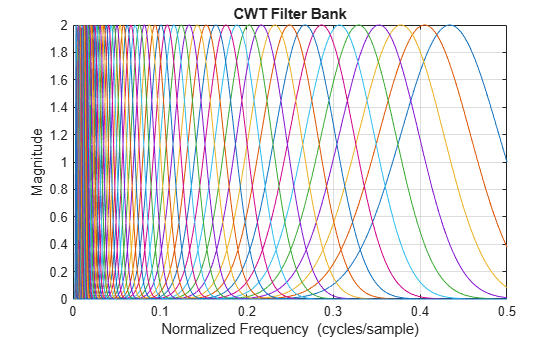Create two sine waves with frequencies of 16 and 64 Hz. The data is sampled at 1000 Hz. Plot the signal.

```Fs = 1e3; t = 0:1/Fs:1-1/Fs; x = cos(2*pi*64*t).*(t>=0.1 & t<0.3)+ ... sin(2*pi*16*t).*(t>=0.5 & t<0.9); plot(t,x) title("Signal") xlabel("Time (s)") ylabel("Amplitude")```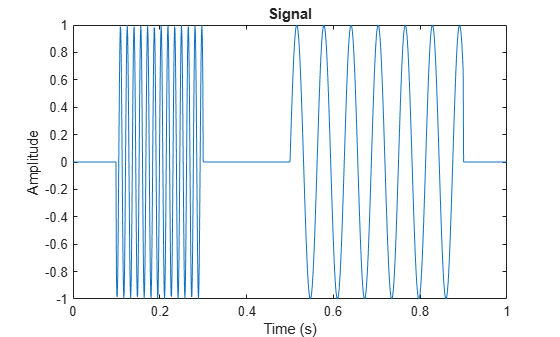Create a CWT filter bank for the signal. Plot the frequency responses of the wavelets in the filter bank.

```fb = cwtfilterbank(SignalLength=numel(t),SamplingFrequency=Fs); figure freqz(fb) title("Frequency Responses — Morse (3,60) Wavelet")```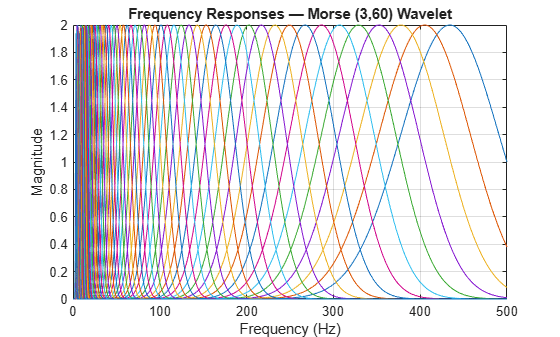The analytic Morse (3,60) wavelet is the default wavelet in the filter bank. The wavelet has a time-bandwidth product equal to 60. Create a second filter bank identical to the first filter bank but instead uses the analytic Morse (3,5) wavelet. Plot the frequency responses of the wavelets in the second filter bank.

```fb3x5 = cwtfilterbank(SignalLength=numel(t),SamplingFrequency=Fs,... TimeBandwidth=5); figure freqz(fb3x5) title("Frequency Responses — Morse (3,5) Wavelet")```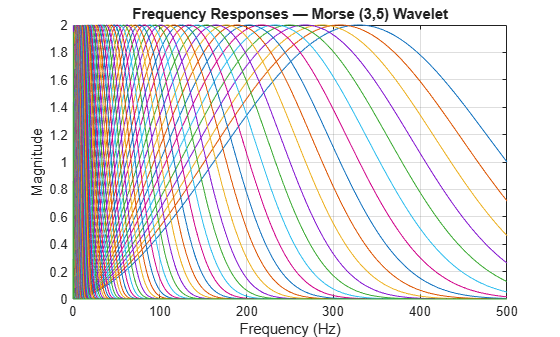Observe that the frequency responses are wider than in the first filter bank. The Morse (3,60) wavelet is better localized in frequency than the Morse (3,5) wavelet. Apply each filter bank to the signal and plot the resulting scalograms. Observe that the Morse (3,60) wavelet has better frequency resolution than the Morse (3,5) wavelet.

```figure cwt(x,FilterBank=fb) title("Magnitude Scalogram — Morse (3,60)")```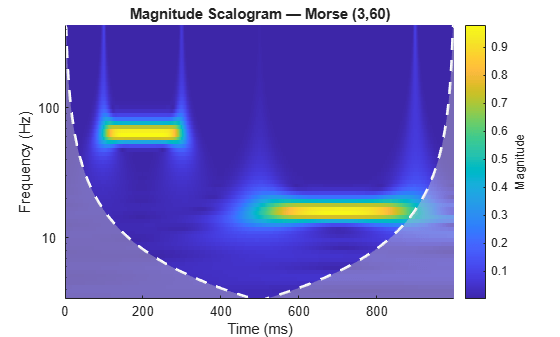```figure cwt(x,FilterBank=fb3x5) title("Magnitude Scalogram — Morse (3,5)")```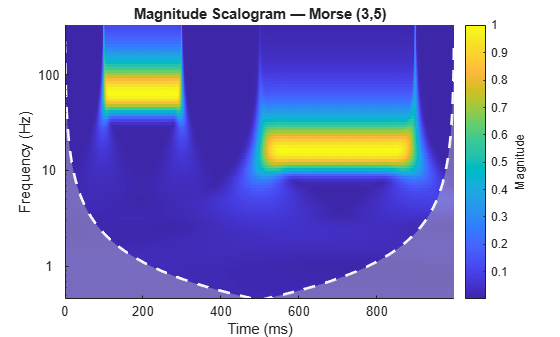This example shows that the amplitudes of oscillatory components in a signal agree with the amplitudes of the corresponding wavelet coefficients.

Create a signal composed of two sinusoids with disjoint support in time. One sinusoid has a frequency of 32 Hz and amplitude equal to 1. The other sinusoid has a frequency of 64 Hz and amplitude equal to 2. The signal is sampled for one second at 1000 Hz. Plot the signal.

```frq1 = 32; amp1 = 1; frq2 = 64; amp2 = 2; Fs = 1e3; t = 0:1/Fs:1; x = amp1*sin(2*pi*frq1*t).*(t>=0.1 & t<0.3)+... amp2*sin(2*pi*frq2*t).*(t>0.6 & t<0.9); plot(t,x) grid on xlabel("Time (sec)") ylabel("Amplitude") title("Signal")```Create a CWT filter bank that can be applied to the signal. Since the signal component frequencies are known, set the frequency limits of the filter bank to a narrow range that includes the known frequencies. To confirm the range, plot the magnitude frequency responses for the filter bank.

```fb = cwtfilterbank(SignalLength=numel(x),SamplingFrequency=Fs,... FrequencyLimits=[20 100]); freqz(fb)```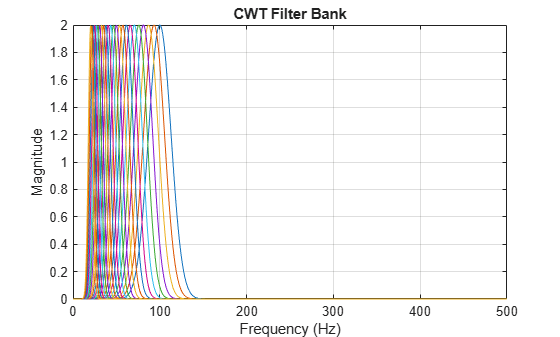Use `cwt` and the filter bank to plot the scalogram of the signal.

`cwt(x,FilterBank=fb)`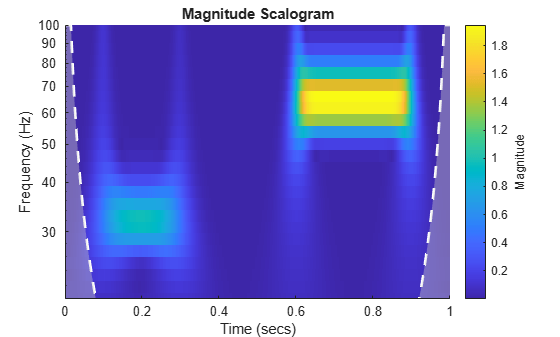Use a data cursor to confirm that the amplitudes of the wavelet coefficients are essentially equal to the amplitudes of the sinusoidal components. Your results should be similar to the ones in the following figure.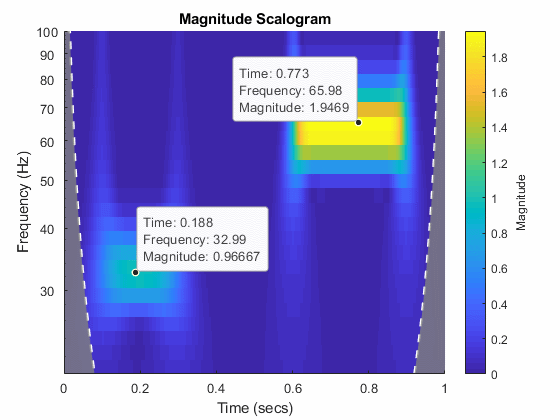This example shows how to vary the time-bandwidth parameter of the generalized Morse wavelet to approximate the analytic Morlet wavelet.

Generalized Morse wavelets are a family of exactly analytic wavelets. Morse wavelets have two parameters, symmetry and time-bandwidth product. You can vary these parameters to obtain analytic wavelets with different properties and behaviors. For additional information, see Morse Wavelets and the references therein.

Load the seismograph data recorded during the 1995 Kobe earthquake. The data are seismograph (vertical acceleration, nm/sq.sec) measurements recorded at Tasmania University, Hobart, Australia on 16 January 1995 beginning at 20:56:51 (GMT) and continuing for 51 minutes at 1 second intervals. Create a CWT filter bank with default settings that can be applied to the data. Use the filter bank to generate the scalogram.

```load kobe fb = cwtfilterbank(SignalLength=numel(kobe),SamplingFrequency=1); cwt(kobe,FilterBank=fb)```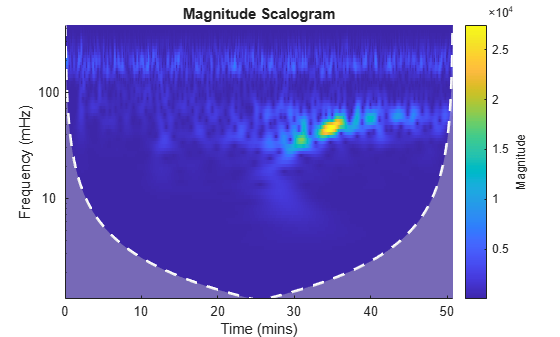The magnitude of the wavelet coefficients is large in the frequency range from 10 mHz to 100 mHz. Create a new filter bank with frequency limits set to these values. Generate the scalogram.

```fb2 = cwtfilterbank(SignalLength=numel(kobe),SamplingFrequency=1,... FrequencyLimits=[1e-2 1e-1]); cwt(kobe,FilterBank=fb2) title("Default Morse (3,60) Wavelet")```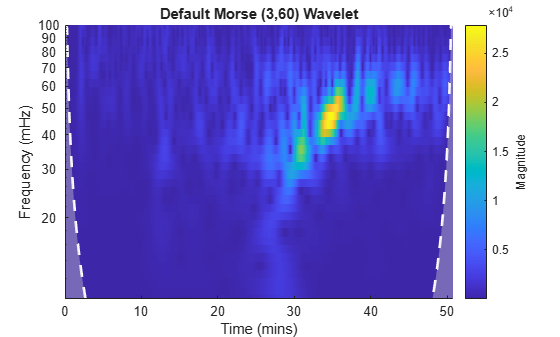By default, `cwtfilterbank` uses the Morse (3,60) wavelet. Create a filter bank using the analytic Morlet wavelet with the same frequency limits. Generate a scalogram and compare with the scalogram generated by the Morse (3,60) wavelet.

```fbMorlet = cwtfilterbank(SignalLength=numel(kobe),SamplingFrequency=1,... FrequencyLimits=[1e-2 1e-1],... Wavelet="amor"); cwt(kobe,FilterBank=fbMorlet) title("Analytic Morlet Wavelet")```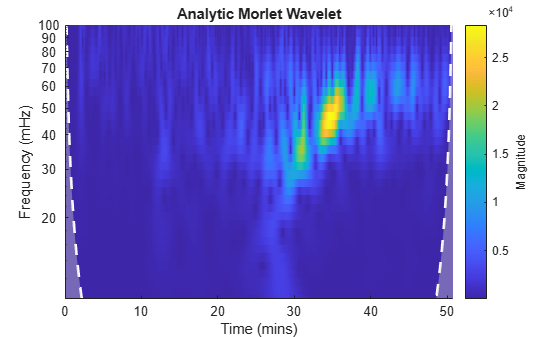The Morlet wavelet is not as well localized in frequency as the (3,60) Morse wavelet. However, by varying the time-bandwidth product, you can create a Morse wavelet with properties similar to the Morlet wavelet.

Create a filter bank using the Morse wavelet with a time-bandwidth value of 30 , with frequency limits as above. Generate the scalogram of the seismograph data. Note there is smearing in frequency nearly identical to the Morlet results.

```fbMorse = cwtfilterbank(SignalLength=numel(kobe),SamplingFrequency=1,... FrequencyLimits=[1e-2 1e-1],... TimeBandwidth=30); cwt(kobe,FilterBank=fbMorse) title("Morse (3,30)")```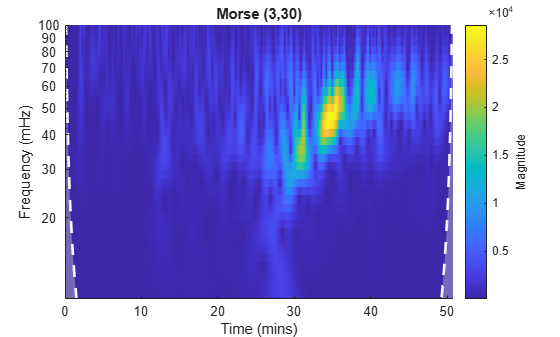Now examine the wavelets associated with the `fbMorlet` and `fbMorse` filter banks. From both filter banks, obtain the wavelet center frequencies, filter frequency responses, and time-domain wavelets. Confirm the center frequencies are nearly identical.

```cfMorlet = centerFrequencies(fbMorlet); [frMorlet,fMorlet] = freqz(fbMorlet); [wvMorlet,tMorlet] = wavelets(fbMorlet); cfMorse = centerFrequencies(fbMorse); [frMorse,fMorse] = freqz(fbMorse); [wvMorse,tMorse] = wavelets(fbMorse); disp(["Number of Center Frequencies: ",num2str(length(cfMorlet))]);```
``` "Number of Center Frequencies: " "34" ```
`disp(["Maximum difference: ",num2str(max(abs(cfMorlet-cfMorse)))]);`
``` "Maximum difference: " "2.7756e-17" ```

Each filter bank contains the same number of wavelets. Choose a center frequency, and plot the frequency response of the associated filter from each filter bank. Confirm the responses are nearly identical.

```wv = 13; figure plot(fMorlet,frMorlet(wv,:)); hold on plot(fMorse,frMorse(wv,:)); grid on hold off title("Frequency Response") xlabel("Frequency") ylabel("Amplitude") legend("Morlet","Morse (3,30)")```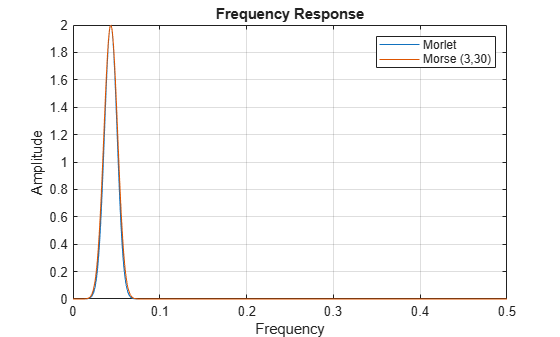Plot the time-domain wavelets associated with the same center frequency. Confirm they are nearly identical.

```figure subplot(2,1,1) plot(tMorlet,real(wvMorlet(wv,:))) hold on plot(tMorse,real(wvMorse(wv,:))) grid on hold off title("Real") legend("Morlet","Morse (3,30)") xlim([-100 100]) subplot(2,1,2) plot(tMorlet,imag(wvMorlet(wv,:))) hold on plot(tMorse,imag(wvMorse(wv,:))) grid on hold off title("Imaginary") legend("Morlet","Morse (3,30)") xlim([-100 100])```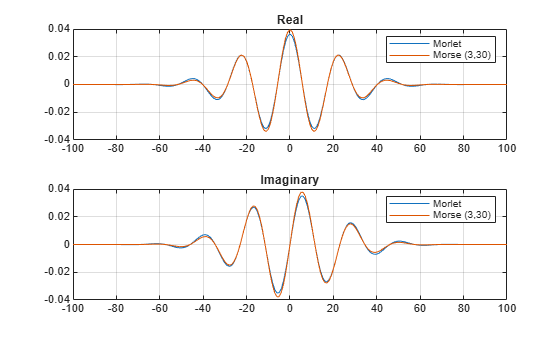This example shows that increasing the time-bandwidth product ${P}^{2}$ of the Morse wavelet creates a wavelet with more oscillations under its envelope. Increasing ${P}^{2}$ narrows the wavelet in frequency.

Create two filter banks. One filter bank has the default `TimeBandwidth` value of 60. The second filter bank has a `TimeBandwidth` value of 10. The `SignalLength` for both filter banks is 4096 samples.

```sigLen = 4096; fb60 = cwtfilterbank(SignalLength=sigLen); fb10 = cwtfilterbank(SignalLength=sigLen,TimeBandwidth=10);```

Obtain the time-domain wavelets for the filter banks.

```[psi60,t] = wavelets(fb60); [psi10,~] = wavelets(fb10);```

Use the `scales` function to find the mother wavelet for each filter bank.

```sca60 = scales(fb60); sca10 = scales(fb10); [~,idx60] = min(abs(sca60-1)); [~,idx10] = min(abs(sca10-1)); m60 = psi60(idx60,:); m10 = psi10(idx10,:);```

Since the time-bandwidth product is larger for the `fb60` filter bank, verify the `m60` wavelet has more oscillations under its envelope than the `m10` wavelet.

```subplot(2,1,1) plot(t,abs(m60)) grid on hold on plot(t,real(m60)) plot(t,imag(m60)) hold off xlim([-30 30]) legend("abs(m60)","real(m60)","imag(m60)") title("TimeBandwidth = 60") subplot(2,1,2) plot(t,abs(m10)) grid on hold on plot(t,real(m10)) plot(t,imag(m10)) hold off xlim([-30 30]) legend("abs(m10)","real(m10)","imag(m10)") title("TimeBandwidth = 10")```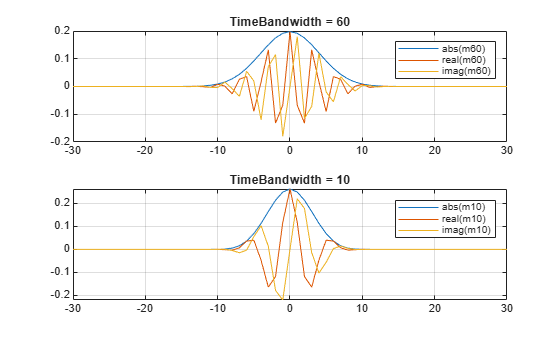Align the peaks of the `m60` and `m10` magnitude frequency responses. Verify the frequency response of the `m60` wavelet is narrower than the frequency response for the `m10` wavelet.

```cf60 = centerFrequencies(fb60); cf10 = centerFrequencies(fb10); m60cFreq = cf60(idx60); m10cFreq = cf10(idx10); freqShift = 2*pi*(m60cFreq-m10cFreq); x10 = m10.*exp(1j*freqShift*(-sigLen/2:sigLen/2-1)); figure plot([abs(fft(m60)).' abs(fft(x10)).']) grid on legend("Time-bandwidth = 60","Time-bandwidth = 10") title("Magnitude Frequency Responses")```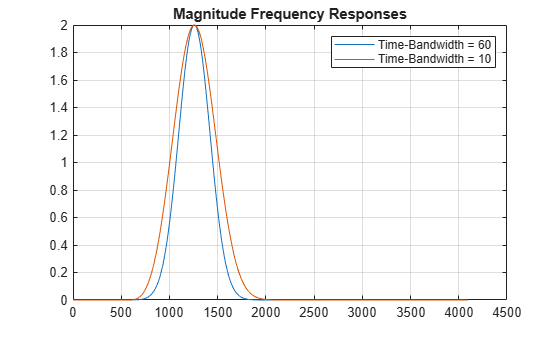This example shows how using a CWT filter bank can improve computational efficiency when taking the CWT of multiple time series.

Create a 100-by-1024 matrix `x`. Create a CWT filter bank appropriate for signals with 1024 samples.

```x = randn(100,1024); fb = cwtfilterbank;```

Use `cwt` with default settings to obtain the CWT of a signal with 1024 samples. Create a 3-D array that can contain the CWT coefficients of 100 signals, each of which has 1024 samples.

```cfs = cwt(x(1,:)); res = zeros(100,size(cfs,1),size(cfs,2));```

Use the `cwt` function and take the CWT of each row of the matrix `x`. Display the elapsed time.

```tic for k=1:100 res(k,:,:) = cwt(x(k,:)); end toc```
```Elapsed time is 0.928160 seconds. ```

Now use the `wt` object function of the filter bank to take the CWT of each row of `x`. Display the elapsed time.

```tic for k=1:100 res(k,:,:) = wt(fb,x(k,:)); end toc```
```Elapsed time is 0.393524 seconds. ```

This example shows how to plot the CWT scalogram in a figure subplot.

Load the speech sample. The data is sampled at 7418 Hz. Plot the default CWT scalogram.

```load mtlb cwt(mtlb,Fs)```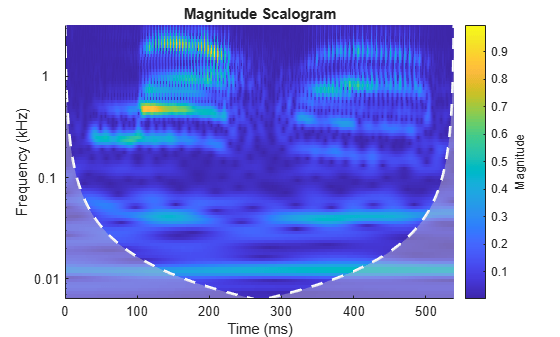Obtain the continuous wavelet transform of the signal, and the frequencies of the CWT.

`[cfs,frq] = cwt(mtlb,Fs);`

The `cwt` function sets the time and frequency axes in the scalogram. Create a vector representing the sample times.

`tms = (0:numel(mtlb)-1)/Fs;`

In a new figure, plot the original signal in the upper subplot and the scalogram in the lower subplot. Plot the frequencies on a logarithmic scale.

```figure subplot(2,1,1) plot(tms,mtlb) axis tight title("Signal and Scalogram") xlabel("Time (s)") ylabel("Amplitude") subplot(2,1,2) surface(tms,frq,abs(cfs)) axis tight shading flat xlabel("Time (s)") ylabel("Frequency (Hz)") set(gca,"yscale","log")```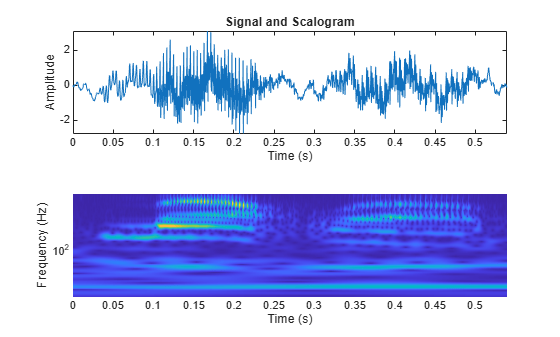## Tips

• The first time you use a filter bank to take the CWT of a signal, the wavelet filters are constructed to have the same datatype as the signal. A warning message is generated when you apply the same filter bank to a signal with a different datatype. Changing datatypes comes with the cost of redesigning or changing the precision of the filter bank. For optimal performance, use a consistent datatype.

• When performing multiple CWTs, for example inside a for-loop, the recommended workflow is to first create a `cwtfilterbank` object and then use the `wt` object function. This workflow minimizes overhead and maximizes performance. See Using CWT Filter Bank on Multiple Time Series.

 Lilly, J. M., and S. C. Olhede. “Generalized Morse Wavelets as a Superfamily of Analytic Wavelets.” IEEE Transactions on Signal Processing. Vol. 60, No. 11, 2012, pp. 6036–6041.

 Lilly, J. M., and S. C. Olhede. “Higher-Order Properties of Analytic Wavelets.” IEEE Transactions on Signal Processing. Vol. 57, No. 1, 2009, pp. 146–160.

 Lilly, J. M. jLab: A data analysis package for Matlab, version 1.6.2. 2016. http://www.jmlilly.net/jmlsoft.html.

 Lilly, J. M. “Element analysis: a wavelet-based method for analysing time-localized events in noisy time series.” Proceedings of the Royal Society A. Volume 473: 20160776, 2017, pp. 1–28. dx.doi.org/10.1098/rspa.2016.0776.